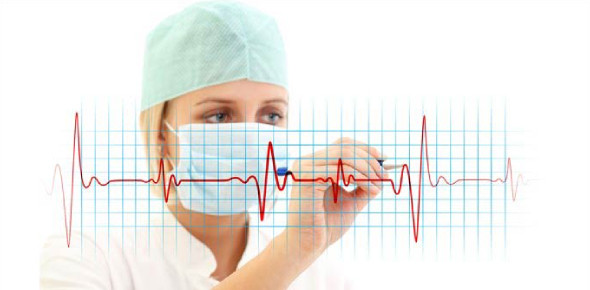# NCCT ECG Certification Review Questions! Quiz

50 Questions | Total Attempts: 4678SettingsIn an exam, there are certain terminologies you must be aware of so that you can get the meaning of the question or have a clue on what it answers can be. With the NCCT ECG certification exam just around the corner, the quiz below is designed to help you review some medical terminologies. Give it a try and keep a look out for part two.

• 1.
AF is an abbreviation for
• A.

A. atrial filter

• B.

B. atrial focus

• C.

C. atrial fibrillation

• D.

D. auto focus

• 2.
• A.

A. attach

• B.

B. sit on top

• C.

C. cut through

• D.

D. separate itself

• 3.
NPO is an abbreviation for
• A.

A. nothing by mouth

• B.

B. nothing put on

• C.

C. non-protein origin

• D.

D. None of the answers are correct

• 4.
NSR is an abbreviation for
• A.

A. normal sinus rhythm

• B.

B. normal sinus rate

• C.

C. non-sinus resonance

• D.

• 5.
• A.

A. cardiac abnormality disease

• B.

B. coronary artery disease

• C.

C. coronary abnormality disease

• D.

D. cardiac artery disorder

• 6.
SOB is an abbreviation for
• A.

• B.

B. serious occlusion / breathing

• C.

C. shortness of breath

• D.

D. None of the answers are correct

• 7.
The medical term that means an obstruction of blood is
• A.

A. hypoxia

• B.

B. angina

• C.

C. ischemia

• D.

D. coagulation

• 8.
A patient who is hypothermic would exhibit a body temperature that is
• A.

A. above normal

• B.

B. below normal

• C.

C. within normal limits

• D.

D. abnormal

• 9.
An axillary temperature is taken
• A.

A. under the tongue

• B.

B. inside the ear

• C.

C. rectally

• D.

D. in the armpit

• 10.
A measurement that is 10mm in length is the same as
• A.

A. 1 liter

• B.

B. 1 centimeter

• C.

C. 1 millimeter

• D.

D. 1 cubic centimeter

• 11.
Pain with sudden onset that is severe in nature is called
• A.

A. acute

• B.

B. chronic

• C.

C. psychosomatic

• D.

• 12.
A patient who is described as afebrile is one who has
• A.

A. a high fever

• B.

B. normal body temperature

• C.

C. been defibrillated

• D.

• 13.
occur(s) when heart muscle cannot get oxygen and the tissue dies
• A.

A. Paroxysmal tachycardia

• B.

B. Myocardial infarction

• C.

C. Cardiac palpitations

• D.

D. Myocarditis

• 14.
When a depolarization wavefront moves perpendicular to a positive electrode, it creates                  on the ECG.
• A.

A. a negative deflection

• B.

B. a positive deflection

• C.

C. electronic noise

• D.

D. equiphasic or isoelectric complexes

• 15.
RBBB is an abbreviation for
• A.

A. right bundle branch block

• B.

B. right branch bundle block

• C.

C. right block of bundle branches

• D.

D. resisting bundle branch block

• 16.
Electrode contact in an ECG can be improved by
• A.

• B.

B. cleaning or drying the skin

• C.

C. using a contact medium

• D.

• 17.
VT is the abbreviation for ventricular
• A.

A. touching

• B.

B. tachyarrhythmia

• C.

C. tachycardia

• D.

D. transition

• 18.
• A.

A. lateral anterior deviation

• B.

B. left anterior deviation

• C.

C. left axis deviation

• D.

D. lateral axis deviation

• 19.
The abbreviation for medicine is
• A.

A. MD

• B.

B. med

• C.

C. medi

• D.

D. min

• 20.
The abbreviation for medical doctor is
• A.

A. DR

• B.

B. LPN

• C.

C. RN

• D.

D. MD

• 21.
Which of these is an abbreviation for hour?
• A.

A. lit

• B.

B. hs

• C.

C. ho

• D.

D. hr

• 22.
The abbreviation for electroencephalogram is
• A.

A. EEG

• B.

B. ECG

• C.

C. EKG

• D.

D. EECG

• 23.
The abbreviation for temperature, pulse and respiration is
• A.

A. temp

• B.

B. tpr

• C.

C. trfc

• D.

D. vtls

• 24.
The abbreviation for at once or immediately is
• A.

A. Rx

• B.

B. asap

• C.

C. stat

• D.

D. std

• 25.
The abbreviation for cardiopulmonary resuscitation is
• A.

A. CBR

• B.

B. CPR

• C.

C. CA

• D.

D. Cath

Related TopicsBack to top